A feature for showing Percentiles based on aggregated results is available in StoryTeller charts. It can be used in customer satisfaction survey for showing the ranges of the all the units in a survey for getting relevant benchmark figures as shown in the example chart below.

The chart shows different questions in the X axis. The black line shows the result of the selected Unit in a company and the stacked columns show the 25, 50, 75 and 100 percentiles of all units in the company. By comparing the black line and the stacked columns the user can see how his/her performance relates to the other units in the company.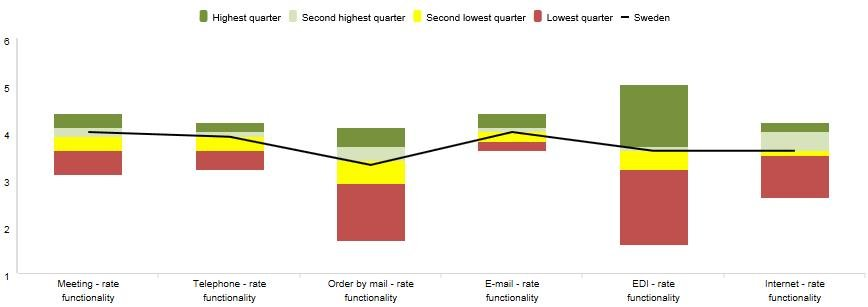The percentile calculation uses aggregated results shown in a chart and visualizes those as percentiles, the calculation is not using respondent level data. The setup is flexible. The number of percentiles shown in the chart, the colors and labels for each percentile are defined during the setup. The image below shows the setup panel of the Percentiles, it is located in the Analysis tab in the chart setup window.As shown in the setup image above the Administrator defines a “Start percentile”. The “Start percentile” is only defined if the selected chart type is an Area, Bar or Column as those types shows ranges of values (Line and Spline types do not visualize result in ranges so the definition of a start percentile is not enabled when those types are selected). To create a chart like the one in the example above the two series have been used:

• Series 1: used for showing the percentiles
• Series 2: used for showing the result of the selected group (the black line).

The original layout of series 1 shows the result of all the units in the company and then the percentile calculation has been applied to visualize the result in percentiles instead of the result per unit. The image below shows the original series 1, how it looked like before the percentile calculation was applied. The chart shows the result of each unit in the company in stacked columns.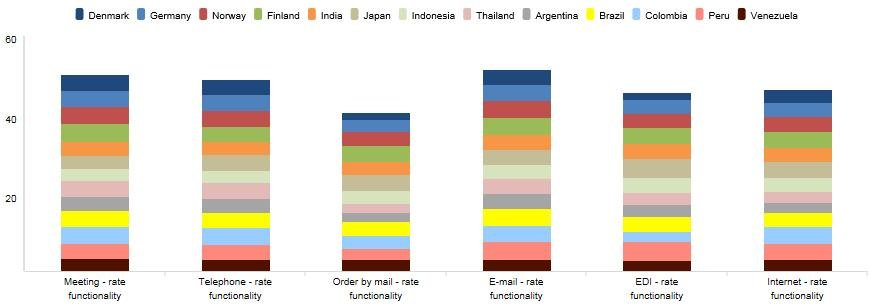The image below shows the percentile setup, as shown 4 percentile ranges are applied, the range from 0-25, the range from 25-50, the range from 50-75 and the range from 75-100.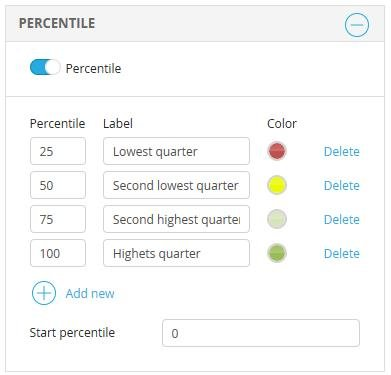The image below shows how series 1 looks like when the percentile calculation is applied, the result per unit has been replaced with the four percentiles.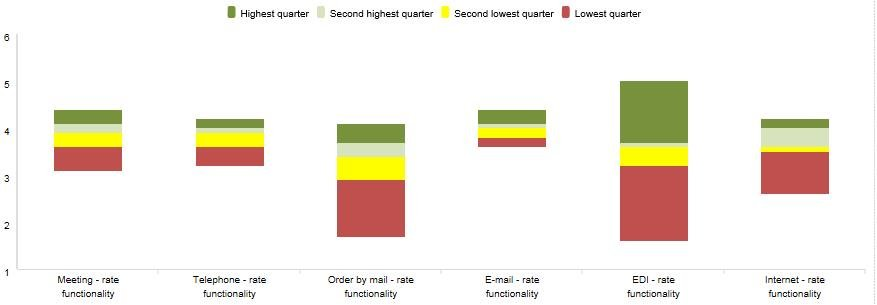The “Start percentile” can be defined when using charts showing results in ranges (columns, bar, and area). The first chart below shows one percentile defined as the 90th percentile, the start value has been set to 0. This means that the column shows the range from the lowest value to the 90th percentile. In the second image below the start value has been set to 10 which means that the column shows the range from the 10th to the 90th percentile.

Example 1.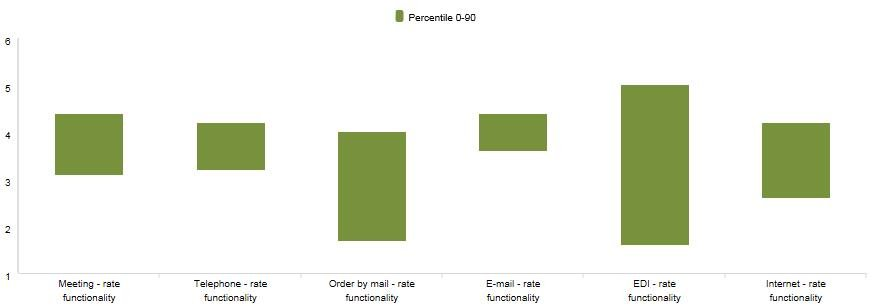Example 2.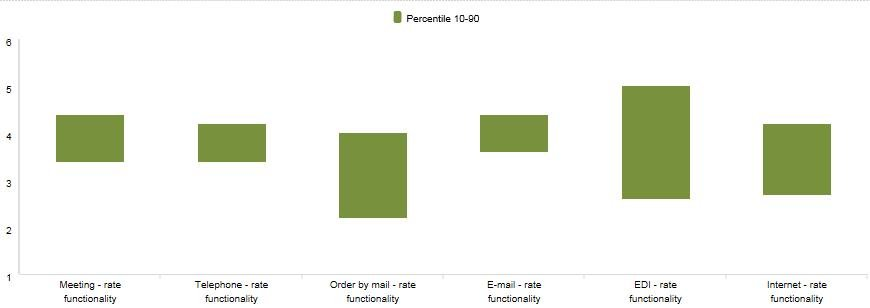The formula shown below is used for calculating the percentile value, it is the same formula as being used in the Cross table tool and also in the Excel software### As an example:

Consider the ordered list {15, 20, 35, 40, 50}, which contains five data values. What is the 40th percentile of this list using this variant method?

First we calculate the rank of the 40th percentile:So, x=2.6, which is between value 2 and 3. Value 2 and 3 in our range are respectively 20 and 35 and the 40th percentile will fall somewhere in between.

Then x=2.6 gives usand. So, the value of the 40th percentile is